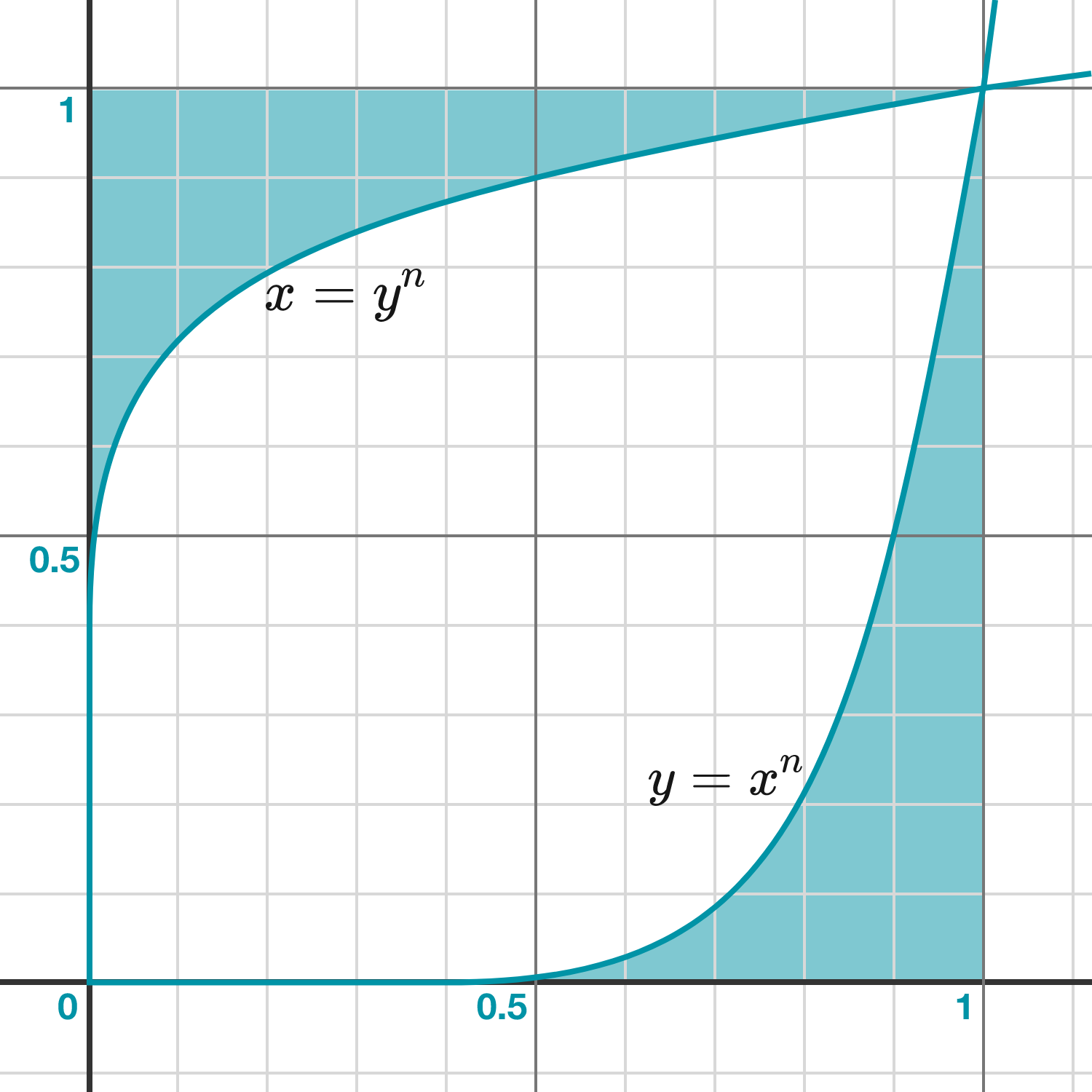Calculus Level 3If the area of the shaded region is $\dfrac1{1009} \text{ unit}^2$ for constant $n$, find $n$.

×